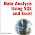## Thursday, February 25, 2010

### Agglomerative Variable Clustering

Lately, I've been thinking about the topic of reducing the number of variables, and how this is a lot like clustering variables (rather than clustering rows). This post is about a method that seems intuitive to me, although I haven't found any references to it. Perhaps a reader will point me to references and a formal name. This method using Pearson correlation and principal components to agglomeratively cluster the variables.

Agglomerative clustering is the process of assigning records to clusters, starting with the records that are closest to each other. This process is repeated, until all records are placed into a single cluster. The advantage of agglomerative clustering is that it creates a structure for the records, and the user can see different numbers of clusters. Divisive clustering, such as implemented by SAS's varclus proc, produces something similar, but from the top-down.

Agglomerative variable clustering works the same way. Two variables are put into the same cluster, based on their proximity. The cluster then needs to be defined in some manner, by combining information in the cluster.

The natural measure for proximity is the square of the (Pearson) correlation between the variables. This is a value between 0 and 1 where 0 is totally uncorrelated and 1 means the values are colinear. For those who are more graphically inclined, this statistic has an easy interpretation when there are two variables. It is the R-square value of the first principal component of the scatter plot.

Combining two variables into a cluster requires creating a single variable to represent the cluster. The natural variable for this is the first principal component.

My proposed clustering method repeatedly does the following:
1. Finds the two variables with the highest correlation.
2. Calculates the principal component for these variables and adds it into the data.
3. Maintains the information that the two variables have been combined.
The attached SAS code (available at sas-var-hierarchical-clustering-v01.sas) does exactly this, although not in the most efficient and robust way. The bulk of the code is a macro, called buildcolumns, that appends the new cluster variables to the data set and maintains another table called columns which has the information about the rows. After I run this code, I can select different numbers of variables using the expression:

proc sql;
....select colname
....from columns
....where counter <= [some number] <>

These variables can then be used for predictive models or visualization purposes.

The inner loop of the code works by doing the following:
1. Calling proc corr to calculate the correlation of all variables not already in a cluster.
2. Transposing the correlations into a table with three columns, two for the variables and one for the correlation using proc transpose.
3. Finding the pair of variables with the largest correlation.
4. Calculating the first principal component for these variables.
5. Appending this principal component to the data set.
6. Updating the columns data set with information about the new cluster.
The data set referred to in the code comes from the companion site for Data Analysis Using SQL and Excel. The code will fail (by running an infinite loop) if any variables are missing or if two variables are exactly correlated.

1.You might be interested in reading:

A data-driven functional projection approach for the selection of feature ranges in spectra with ICA or cluster analysis
Catherine Krier, Fabrice Rossi, Damien François and Michel Verleysen
Chemometrics and Intelligent Laboratory Systems, Elsevier, Vol. 91, No. 1 (15 March 2008), pp. 43-53.

http://www.dice.ucl.ac.be/~verleyse/papers/cils08ck.pdf

and the references therein

2.Sas Proc VarClus

3.PROC VARCLUS is specifically my inspiration for thinking about an agglomerative approach to clustering variables. PROC VARCLUS implements various divisive methods, where all the variables are included in a single cluster, and this gets broken into smaller clusters. I realize that when I first wrote this post, I used the term "hierarchical" when I should have used the term "agglomerative"; I have since fixed that.

4.If your goal is to reduce the number of features, I don't see why you are using first principle of component. Although you only use first principle, it is a linear combination of the two variables. So in the actual fact you have not reduced the number of variables.

5.How is this an improvement from performing Pricipal Component Analysis for all the variables and considering only the first n Principal components?

Your comment will appear when it has been reviewed by the moderators.